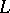# Björling problem

(diff) ← Older revision | Latest revision (diff) | Newer revision → (diff)
A problem in the theory of minimal surfaces (cf. Minimal surface), which is to find the minimal surface passing through a given non-closed analytic curve, with given tangent planes along. Björling's problem on minimal surfaces is the analogue of the Cauchy problem of differential equations. The problem was posed and solved by E.G. Björling . A solution of this problem always exists, is unique and is explicitly expressed by the Schwarz formula for minimal surfaces. The solution of Björling's problem always permits one to find the minimal surface whenever one of its geodesic lines or one of its asymptotic lines or one of its lines of curvature is known. If the given curve is planar and is a geodesic on the minimal surface which is desired to be found, then the plane of the curvewill be a plane of symmetry of the minimal surface.